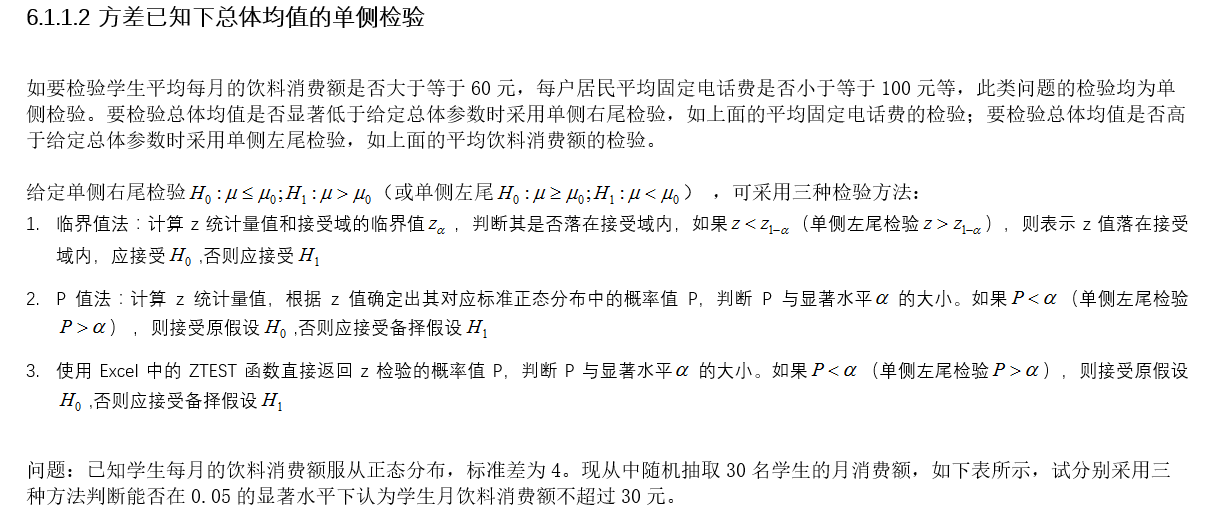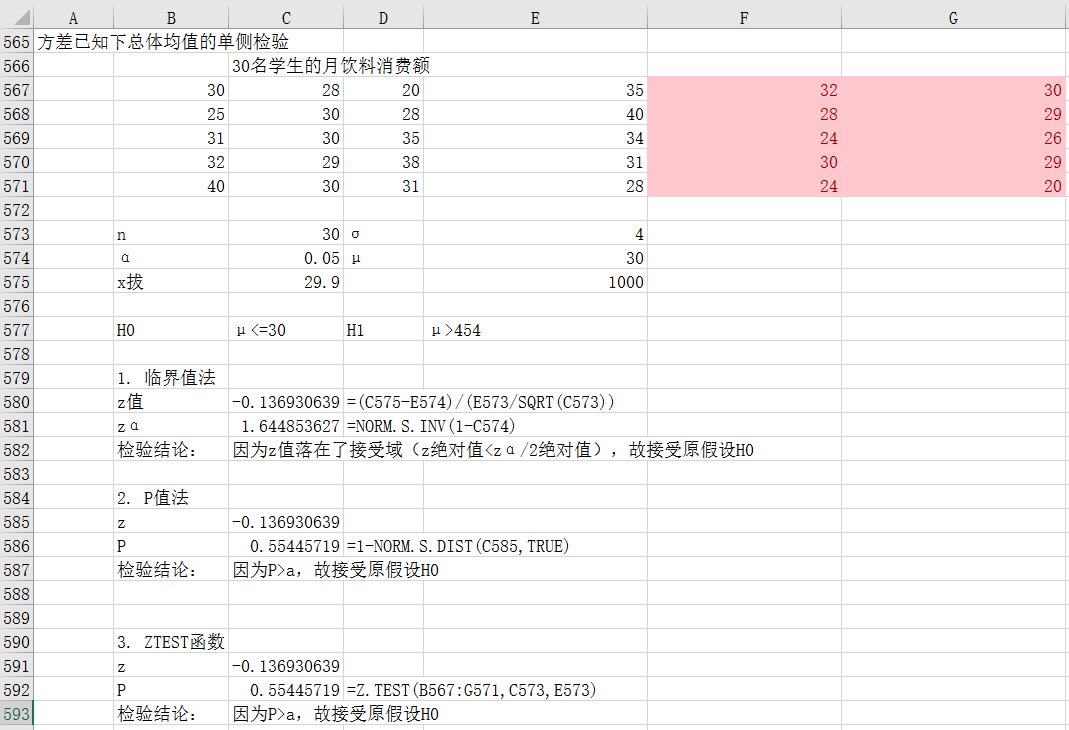• 设XXX和YYY相互独立且XXX~N(μ1,σ12)N(\mu_1,\sigma_1^2)N(μ1​,σ12​)，YYY~N(μ2,σ22)N(\mu_2,\sigma_2^2)N(μ2​,σ22​)，其中σ12\sigma_1^2σ12​和σ22\sigma_2^2σ22​是已知的。来自XXX和YYY的容量分别...
设$X$和$Y$相互独立且$X$~$N(\mu_1,\sigma_1^2)$，$Y$~$N(\mu_2,\sigma_2^2)$，其中$\sigma_1^2$和$\sigma_2^2$是已知的。来自$X$和$Y$的容量分别为$n_1$和$n_2$的样本均值为$\overline{X}$，$\overline{Y}$。对显著水平$\alpha$，检验假设$H_0:\mu_1-\mu_2\geq\delta$（或$H_0:\mu_1-\mu_2\leq\delta$）。由于检验统计量$\frac{\overline{X}-\overline{Y}-\delta}{\sqrt{\sigma_1^2/n_1+\sigma_2^2/n_2}}$~$N(0,1)$，所以，我们可以用函数ztestL或ztestR来计算上述的单侧假设$H_0$。
例1某制造厂声称，其制造的线A的平均张力比线B至少强120N，为证实其说法，在同样情况下测试两种线各50条，线A的平均张力$\overline{x}=867N$，线B的平均张力$\overline{y}=778N$。假设A线和B线的张力分别服从$N(\mu_1,62.8^2)$和$N(\mu_2, 56.1^2)$。取显著水平$\alpha=0.05$，试检验制造厂家的说法。
解： 按题意，需对假设
$H_0:\mu_1-\mu_2\geq120, H_1:\mu_1-\mu_2<120$
作左侧检验，下列代码完成本例计算。
import numpy as np									#导入numpy
xmean=867                                       	#样本均值
ymean=778                                       	#样本均值
xsigma2=62.8**2                                 	#总体方差
ysigma2=56.1**2                                 	#总体方差
delta=120                                       	#总体均值假设值
n1=50                                           	#样本容量
n2=50                                           	#样本容量
alpha=0.05                                      	#显著水平
Z=(xmean-ymean-delta)/np.sqrt(xsigma2/n1+ysigma2/n2)#检验统计量值
accept=ztestL(Z,alpha) 								#计算假设的左侧检验
print('mu1-mu2>=%d is %s'%(delta, accept))

第2~9行按题面设置各项数据。第10行计算检验统计量值$\frac{\overline{x}-\overline{y}-\delta}{\sqrt{\sigma_1^2/n_1+\sigma_2^2/n_2}}$为Z，第11行调用函数ztestL计算左侧检验。运行程序，输出
mu1-mu2>=120 is False.

表示拒绝假设$H_0:\mu_1-\mu_2\geq120$，即厂家所言不实。
例2用两种方法（A和B）测定冰自-0.72$^{\circ}C$转变为0$^{\circ}C$的水的融化热（以cal/g计）测得以下数据：
$\text{方法A：}79.98,80.04,80.02,80.04,80.03,80.03,80.04,79.97,\\ 80.05,80.03,80.02,80.00,80.02\\ \text{方法B：}80.02,79.94,79.98,79.97,79.97,80.03,79.95,79.97$
设A、B方法测定的数据分别服从$N(\mu_1, 0.024^2)$和$N(\mu_2, 0.034^2)$。对$\alpha=0.05$的显著水平，检验假设
$H_0:\mu_1-\mu_2\leq0, H_1:\mu_1-\mu_2>0.$
解： 下列代码完成本例计算。
import numpy as np												#导入numpy
x=np.array([79.98,80.04,80.02,80.04,80.03,80.03,80.04,			#样本数据
79.97,80.05,80.03,80.02,80.00,80.02])
y=np.array([80.02,79.94,79.98,79.97,79.97,80.03,79.95,79.97])	#样本数据
xmean=x.mean()													#样本均值
ymean=y.mean()													#样本均值
s12=0.024**2													#总体方差
s22=0.031**2													#总体方差
n1=x.size														#样本容量
n2=y.size														#样本容量
alpha=0.05														#显著水平
Z=(xmean-ymean)/np.sqrt(s12/n1+s22/n2)							#检验统计量值
accept=ztestR(Z, alpha)											#右侧检验
print('mu1-mu<=0 is %s'%accept)

2~11行按题面设置个数据项，第12行计算检验统计量值$\frac{\overline{x}-\overline{y}}{\sqrt{\sigma_1^2/n_1+\sigma_2^2/n_2}}$为Z（注意，此处$\delta$为0），第13行调用ztestR对假设$H_0:\mu_1-\mu_2\leq0$作右侧检验。运行程序，输出
mu1-mu<=0 is False

表示拒绝假设$H_0:\mu_1-\mu_2\leq0$，即认为方法A比方法B测得的融化热要大。
写博不易，敬请支持：
如果阅读本文于您有所获，敬请点赞、评论、收藏，谢谢大家的支持！
返回《导引》


展开全文• 设XXX和YYY相互独立且XXX~N(μ1,σ12)N(\mu_1,\sigma_1^2)N(μ1​,σ12​)，YYY~N(μ2,σ22)N(\mu_2,\sigma_2^2)N(μ2​,σ22​)，其中σ12\sigma_1^2σ12​和σ22\sigma_2^2σ22​是已知的。来自XXX和YYY的容量分别...
设$X$和$Y$相互独立且$X$~$N(\mu_1,\sigma_1^2)$，$Y$~$N(\mu_2,\sigma_2^2)$，其中$\sigma_1^2$和$\sigma_2^2$是已知的。来自$X$和$Y$的容量分别为$n_1$和$n_2$的样本均值为$\overline{X}$，$\overline{Y}$。对显著水平$\alpha$，检验双侧假设$H_0:\mu_1-\mu_2=\delta,H_1:\mu_1-\mu_2\not=\delta$。由于检验统计量$\frac{\overline{X}-\overline{Y}-\delta}{\sqrt{\sigma_1^2/n_1+\sigma_2^2/n_2}}$~$N(0,1)$，与利用ztestL或ztestR计算已知总体方差计算两总体均值差单侧假设的Z检验相仿，我们可以用函数ztest2计算双侧假设$H_0:\mu_1-\mu_2=\delta$的检验。
例1设甲、乙两厂生产同型号的灯泡，其寿命$X$，$Y$分别服从正态分布$N(\mu_1,\sigma_1^2),\\N(\mu_2, \sigma_2^2)$，已知它们寿命的标准差分别为84h和96h，现从两厂生产的灯泡中各取60只，测得灯泡的平均寿命甲厂为1295h，乙厂为1230h。在显著水平$\alpha=0.05$下，问能否认为两厂生产的灯泡寿命有无显著差别？
解： 按题意，需对假设
$H_0:\mu_1-\mu_2=0, H_1:\mu_1-\mu_2\not=0$
作显著水平为$\alpha=0.05$的双侧检验。下列代码完成本例计算。
import numpy as np								#导入numpy
xmean=1295										#样本均值
ymean=1230										#样本均值
xsigma2=84**2									#总体方差
ysigma2=96**2									#总体方差
n1=60											#样本容量
n2=60											#样本容量
alpha=0.05										#显著水平
Z=(xmean-ymean)/np.sqrt(xsigma2/n1+ysigma2/n2)	#检验统计量值
accept=ztest2(Z, alpha)							#双侧假设Z检验
print('mu1-mu2=0 is %s.'%accept)

第2~8行按题面设置各项数据，第9行计算检验统计量值$\frac{\overline{X}-\overline{Y}-\delta}{\sqrt{\sigma_1^2/n_1+\sigma_2^2/n_2}}$为Z（注意，此时$\delta=0$），第10行调用函数ztest2检验双侧假设$H_0:\mu_1-\mu_2=0$。运行程序，输出
mu1-mu2=0 is False.

表示拒绝假设$H_0:\mu_1-\mu_2=0$，即两厂生产的灯泡寿命有显著差别。
例2 全市高三学生进行数学毕业会考，随机抽取10名男生和8名女生的会考成绩如下：
$\text{男生：}65,72,89,56,79,63,92,48,75,81\\ \text{女生：}78,69,65,61,54,87,51,67$
若男生的会考成绩$X$~$N(\mu_1, 10^2)$，女生的会考成绩$Y$~$N(\mu_2,9.5^2)$，试问男生和女生的平均成绩是否相同（$\alpha=0.05$）？
解： 按题意，需对双侧假设
$H_0:\mu_1-\mu_2=0, H_1:\mu_1-\mu_2\not=0$
作Z检验。下列代码完成本例计算。
import numpy as np#导入numpy
x=np.array([65, 72, 89, 56, 79, 63, 92, 48, 75, 81])#样本数据
y=np.array([78, 69, 65, 61, 54, 87, 51, 67])		#样本数据
xmean=x.mean()										#样本均值
ymean=y.mean()										#样本均值
xsigma2=10**2										#总体方差
ysigma2=9.5**2										#总体方差
n1=x.size											#样本容量
n2=y.size											#样本容量
alpha=0.05											#显著水平
Z=(xmean-ymean)/np.sqrt(xsigma2/n1+ysigma2/n2)		#检验统计量值
accept=ztest2(Z, alpha)								#双侧假设Z检验
print('mu1-mu2=0 is %s.'%accept)

第11行计算检验统计量值$\frac{\overline{X}-\overline{Y}-\delta}{\sqrt{\sigma_1^2/n_1+\sigma_2^2/n_2}}$为Z（注意，此时$\delta=0$），第12行调用函数ztest2检验双侧假设$H_0:\mu_1-\mu_2=0$。运行程序，输出
mu1-mu2=0 is True.

表示接受假设$H_0:\mu_1-\mu_2=0$，即男女生的平均成相同。
写博不易，敬请支持：
如果阅读本文于您有所获，敬请点赞、评论、收藏，谢谢大家的支持！
返回《导引》


展开全文• 小样本，总体为正态分布，总体方差已知，z检验 小样本，总体为正态分布，总体方差未知，用样本方差代替总体方差，t检验 小样本，总体不服从正态分布，将样本量增加到30以上 总体方差检验 总体服从正态分布...
假设检验

1.一个总体参数的检验

总体均值的检验（大样本情况下，样本均值的抽样分布近似服从正态分布）

大样本，总体方差已知，z检验
大样本，总体方差未知，用样本方差代替总体方差，z检验
小样本，总体为正态分布，总体方差已知，z检验
小样本，总体为正态分布，总体方差未知，用样本方差代替总体方差，t检验
小样本，总体不服从正态分布，将样本量增加到30以上
总体方差检验

总体服从正态分布，样本量无论大小，使用卡方检验
2.两个总体参数的检验

两个总体均值之差的检验

独立大样本的检验，方差已知，z检验
独立大样本的检验，方差未知，样本方差代替总体方差，z检验
独立小样本的检验，需要假定总体正态分布，方差已知，z检验
独立小样本的检验，需要假定总体正态分布，方差未知但相等，样本方差代替总体方差，t检验
独立小样本的检验，需要假定总体正态分布，方差未知且不等，t检验
两个总体方差比的检验

样本方差的比值检验，与1相比较，F检验

展开全文• “如要检验学生平均每月的饮料消费额是否大于等于60元，每户居民平均固定电话费是否小于等于100元等，此类问题的检验均为单侧检验。” 解答： 另外， 第一次使用ZTEST函数，比较新鲜，补充...
“如要检验学生平均每月的饮料消费额是否大于等于60元，每户居民平均固定电话费是否小于等于100元等，此类问题的检验均为单侧检验。”解答：另外，

第一次使用ZTEST函数，比较新鲜，补充说明一下。
“For a supplied hypothesized sample mean and a supplied set of values, the Excel Ztest function calculates the one-tailed probability value of the Z-Test.
I.e. the function returns the probability that the supplied hypothesized sample mean is greater than the mean of the supplied data values.
The syntax of the Ztest function is:
ZTEST( array, x, [sigma] )
where the function arguments are:
array-The set of values against which the hypothesized sample mean is to be tested.
x-The hypothesized sample mean.
[sigma]-An optional argument that represents the population standard deviation, if this is known.
If omitted, the calculation uses the sample standard deviation.”

感觉能看懂了，不错！


展开全文• “两样本总体均值之差的假设检验也是经常需要面对的问题，如需要检验经过培训后打字员的打字平均速度是否显著提高，需要检验两个营业部日平均销售额是否显著不同等。”
• “假设检验又称统计假设检验（注：显著性检验只是假设检验中最常用的一种方法），是一种基本的统计推断形式，也是数理统计学的一个重要的分支，用来判断样本与样本，样本与总体的差异是由抽样误差引起还是本质差别...
• 独立性检验在统计教学场合可以替代为 T 检验、F 检验，在研究应用场合应当替代为Odds Ratio置信区间的Fisher检验、或者独立两样本均值之差置信区间（连续性校正的）z 检验或者T 检验。2×2设计的独立性检验是否等价...
• 作者：徐涛，19年应届毕业生，专注于珊瑚礁研究，喜欢用R各种清洗数据。知乎：... 30)，总体标准差σ未知的正态分布。T检验是用t分布理论来推论差异发生的概率，从而比较两个平均数的差异是否显...
• 总体X∼N(μ,σ2),μ,σ2X\sim N(\mu, \sigma^2), \mu, \sigma^2X∼N(μ,σ2),μ,σ2未知，X1,...,XnX_...方差的假设检验也可分为三类：双边检验，左侧检验和右侧检验。 双边检验 假设检验的形式： H0:σ2=σ02H1:σ2
• AB test系列文章目录：冒烟指数：21.AB test(1)初识AB test​zhuanlan.zhihu.com冒烟指数：21.... (3)假设检验基础​zhuanlan.zhihu.com冒烟指数：21.(4)假设检验有哪几类​zhuanlan.zhihu.com冒烟指数：21.（5）AB...
• 开篇引例：某健身俱乐部欲根据往年的会员情况，制定2006年的会员发展营销策略。主管经理估计俱乐部会员的平均年龄是35岁，其中25～35岁的会员占总人数的70%。...统计学是通过假设检验的方法来解决上...统计学
• “对于来自两个总体的样本，我们可以用两个样本方差的比进行分析”。 解答：
• 假设检验 总体均值检验 方差已知 方差未知 步骤 1.总体方差已知 双侧检验与单侧检验 拒绝域和显著性水平 双侧检验 左侧检验 案例 推导 分析: 因为要验证的是新系统有效,及消费超过1700元 那么备择假设...统计学
• 文章目录单个正态总体均值检验均值已知方差未知的单样本t检验双样本的t检验正态总体方差检验二项分布的假设检验 单个正态总体均值检验 library(BSDA) a <- c(8.05,8.15,8.2,8.1,8.25) z.test(x=a,mu=8,...
• 判断一样本所代表的背景总体与理论正态分布是否没有显著差异的检验。 方法一 概率密度曲线比较法 看样本与正太分布概率密度曲线的拟合程度，R代码如下： [plain] view plaincopy norm_...
• 单个正态总体均值检验 (1)sigma已知的u检验（Z检验） #######Z检验 ...#单正态总体方差已知 from scipy import stats from statsmodels.stats import weightstats as stests def test_z_s...数据分析 统计学
• 传送：随机变量概率分布函数汇总-离散型分布+连续型分布 ... 假设检验-两服从正态分布的独立总体均值检验 一、单样本方差检验-需服从正态分布  chisq.var.test=function(x,var,mu=Inf,altern...
• 总体均值的假设检验就是已知了一个均值的先验值，然后根据实验获取的数据对这个值进行验证是否接受它。根据是否已知总体的方差，又可细分为两种类型：方差已知和方差未知。 1. 方差已知的 在方差已知的情况下，检验...数据分析
• 方差分析 在研究一个（或多个）分类型自变量与一个数值型因变量之间的关系时，方差分析就是其中主要...表面上看，方差分析是检验多个总体均值是否相等的统计分析方法，但本质上它所研究的是分类型自变量对数值型因...
• 2. 教材中只提供了TTEST和数据分析之t-检验: 成对双样本均值分析两种方法，主要是借助函数和宏命令来实现的。初学者很难将理论讲解与这些工具关联起来，也就是说，不知道函数即宏命令的结果是怎么算出
• 传送：随机变量概率分布函数汇总-离散型分布+连续型分布 关注的结果变量为连续型组间比较(两组数据必须是独立的)，并...假设条件：X,Y是两个独立的正态总体，，X1,X2...Xn是来自X的样本，Y1,Y2...Yn是来自Y的样本...
• ## 方差检验

千次阅读 2018-08-27 10:22:24
一个总体方差假设检验的汇总 二、两个总体方差的统计推断 两个总体方差假设检验的汇总 三、多个总体比例的相等性的检验 三个或多个相等性的χ2检验 多重比较方法 四、独立性检验 利用样本数据检验两......